# How to use Graphviz## 1. Brief introduction

Graphviz is a visual graphics tool open sourced by AT&T Research and Lucent Bell Labs, which can be easily used to draw structured graph networks and supports multiple output formats. Graphviz input is a drawing script written in dot language. By parsing the input script, it analyzes the points, edges and subgraphs in it, and then draws according to the attributes. Graphviz layouts describe graphs in a simple text language and make diagrams in useful formats such as images and SVG for web pages; PDF and Postscript for placement in other documents or for display in an interactive graph browser.

## 2. Installation

• I was using language bird document comes with text in a drawing to use Graphviz.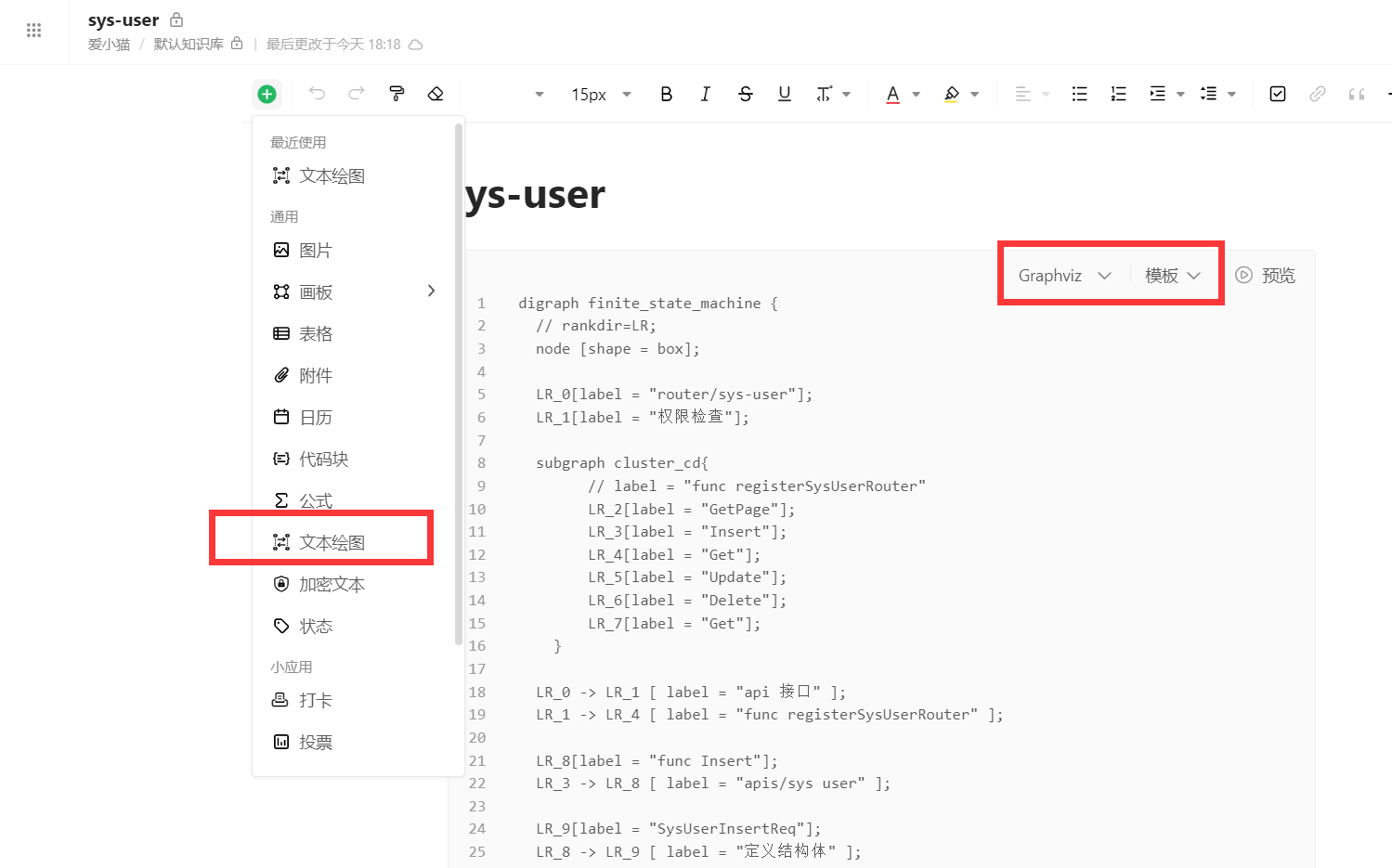• If you want to learn about Graphviz tools, you can also go to Graphviz official website download. After installing according to the installation wizard, remember to add the bin directory of the Graphviz tool to the environment variable PATH. For example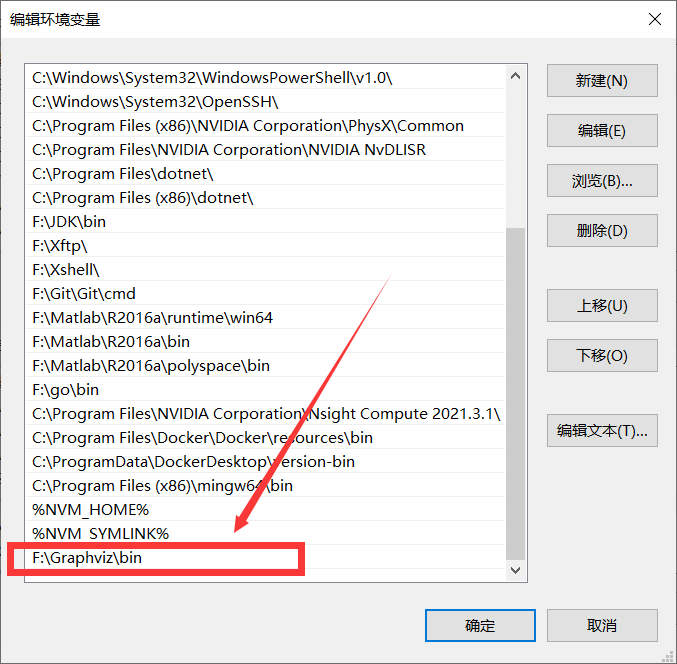Next, test whether the installation is successful, open the cmd command window, enter the command `dot -version` , the following figure appears to prove that the installation was successful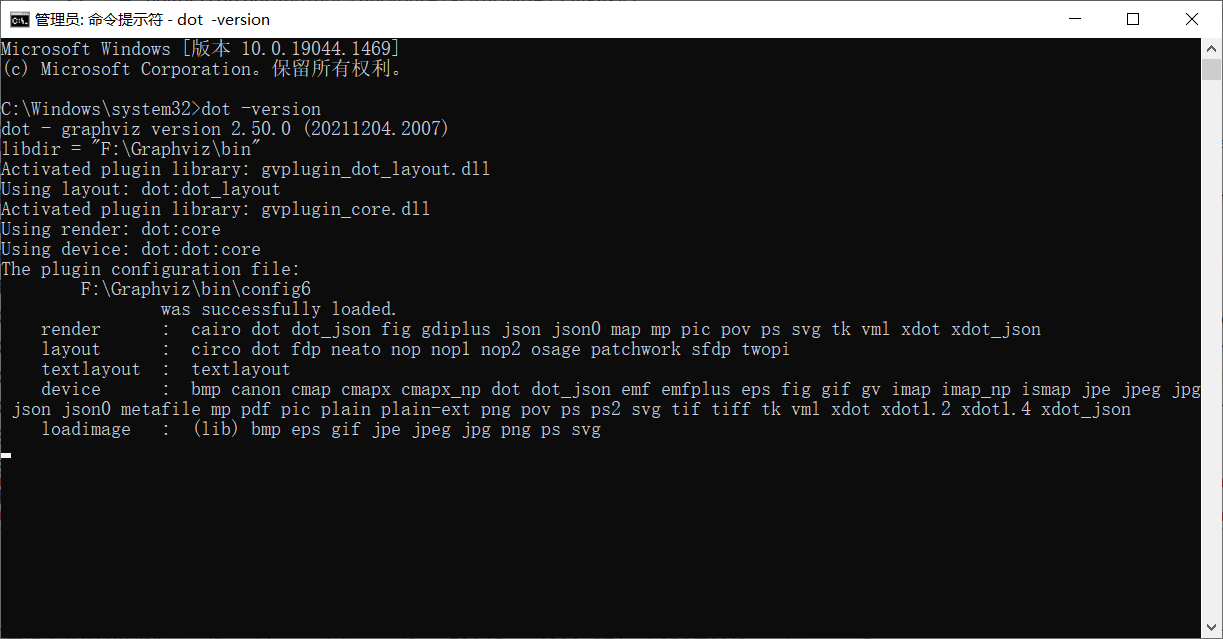## 3. Use

Next is the use of Graphviz language bird
This is the detailed Grapviz usage syntax introduction

### 3.1. Simple use

#### 3.1.1 Undirected graphs

``````graph {
a -- b;
b -- c;
a -- c;
d -- c;
e -- c;
e -- a;
}``````

Effect picture: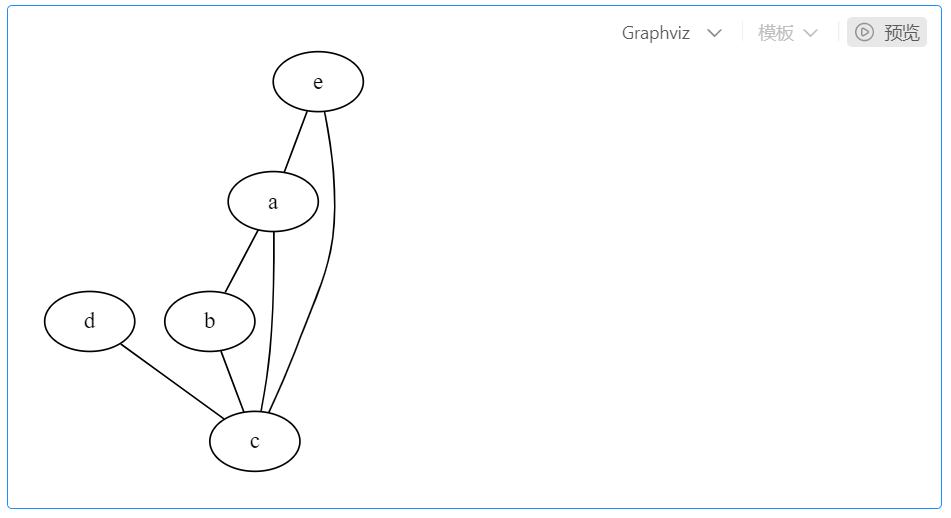#### 3.1.2 Directed Graph

``````digraph {
a -> b;
b -> c;
}``````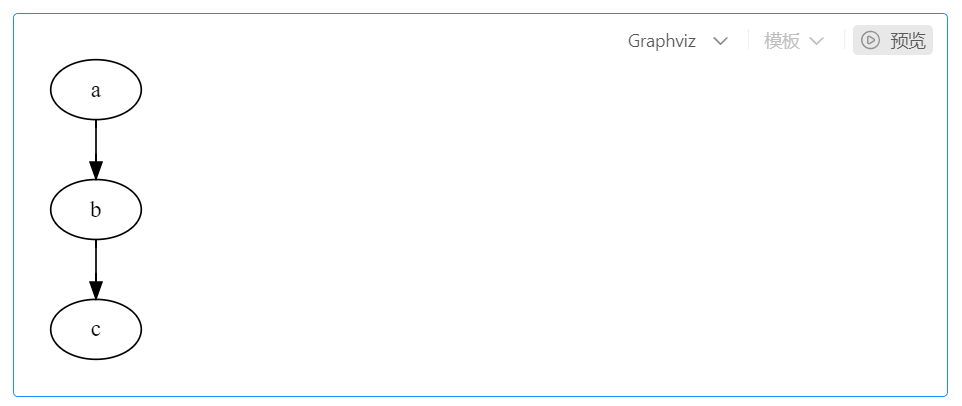### 3.2. Slightly more complicated usage

#### 3.2.1 With labels

``````digraph {
player[label = "player"];
game[label = "game"];
player -> game[label = "play"]
}``````

Effect picture: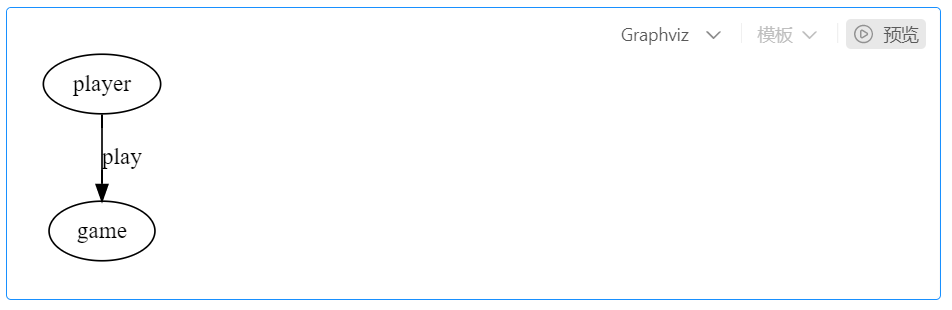#### 3.2.2 Different colors

``````digraph {
player[label = "player", color = Blue, fontcolor = Red, fontsize = 24, shape = box];
game[label = "game", color = Red, fontcolor = Blue, fontsize = 24, shape = ellipse];
player -> game[label = "play"]
}``````

Effect picture: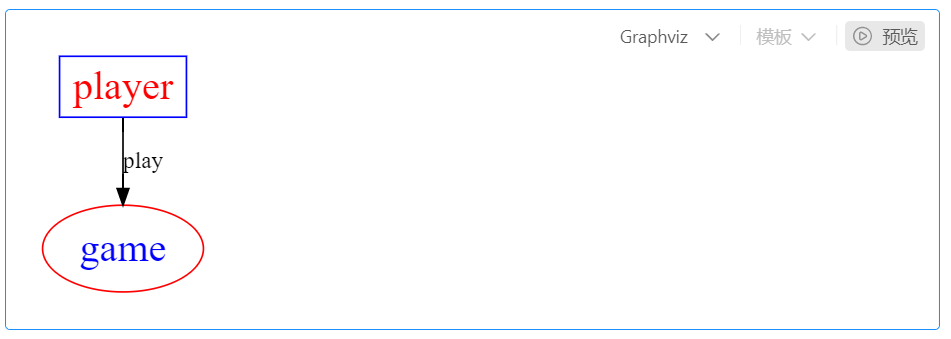#### 3.2.3 Shape

For details, see official document

#### 3.2.4 Insert picture

``````digraph {
c[shape = none, image = "./pic.png"]
a -> b -> c;
c -> d;
}``````

Effect picture: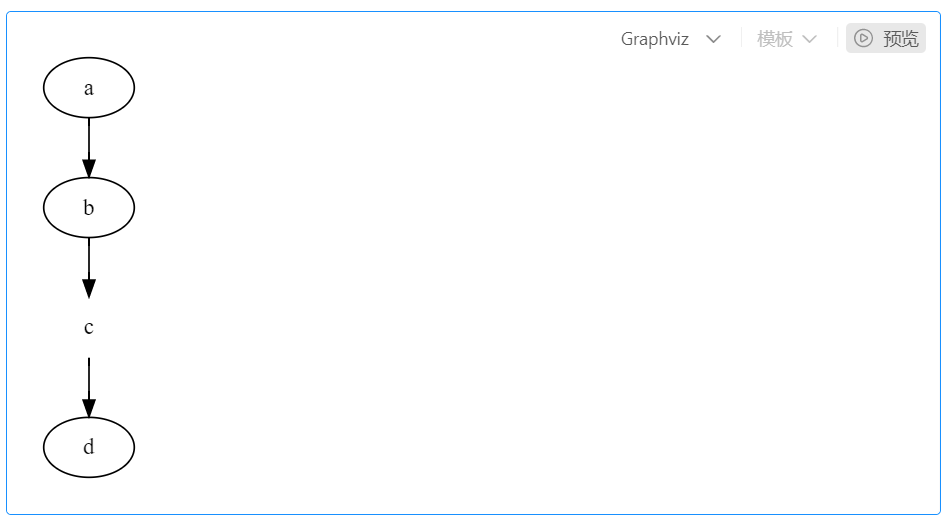#### 3.2.5 Unify Nodes and Connections

``````digraph {
node[shape = box]
edge[style = "dashed"]
c[shape = none]
a -> b -> c;
c -> d;
}``````

Effect picture: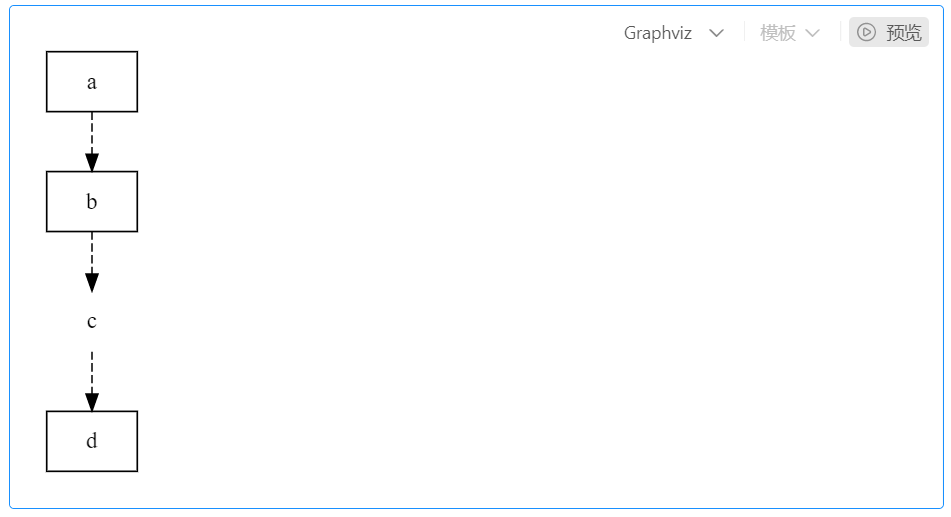#### 3.2.6 Subviews

``````digraph {
label = visitNet
rankdir = LR
node[color = Red, fontsize = 24, shape = box]
edge[color = Blue, style = "dashed"]
user[style = "filled", color = "yellow", fillcolor = "chartreuse"]
subgraph cluster_cd{
label = "server and browser"
bgcolor = yellow;
browser -> server
}
user -> computer;
computer -> browser;
}``````

Effect picture: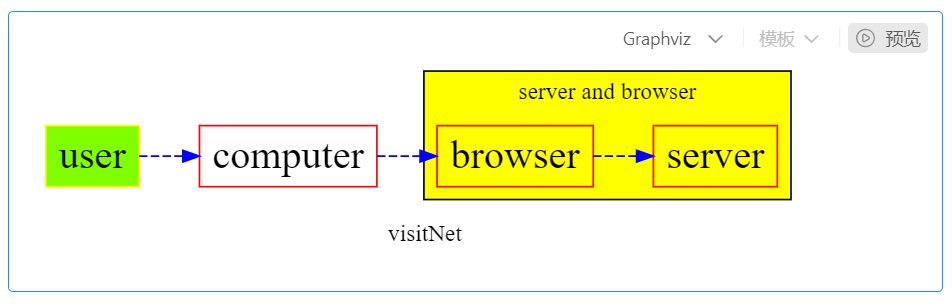#### 3.2.7 Structure View

``````digraph {
node[shape = record];
struct1[label = "<f0> left|<f1> mid&#92; dle|<f2> right"];
struct2[label = "<f0> one|<f1> two"];
struct3[label = "hello&#92;nworld | {b|{c|<here> d|e}|f}|g|h"];
struct1:f1 -> struct2:f0;
struct1:f2 -> struct3:here;
}``````

Effect picture: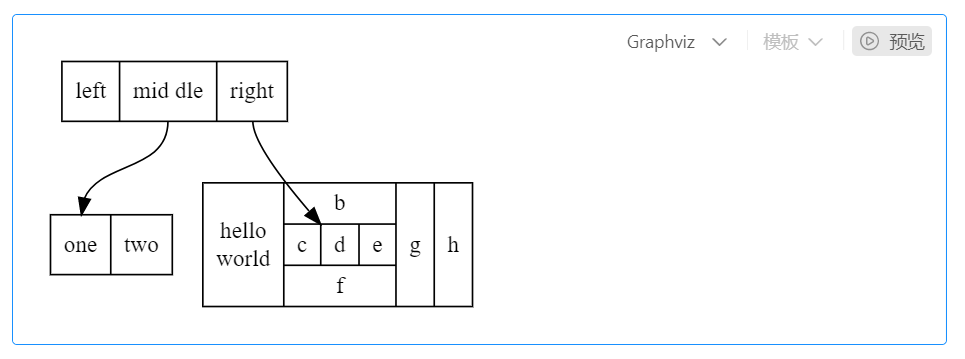#### 3.2.8 Tree structure

``````digraph tree {
fontname = "PingFang-SC-Light"
fontsize = 24
node[shape = "plaintext"]

1 -> 2;
1 -> 3;
2 -> 4;
2 -> 5;
3 -> 6;
3 -> 7;
4 -> 8;
4 -> 9;
5 -> 10;
5 -> 11;
6 -> 12;
6 -> 13;
7 -> 14;
7 -> 15;
}``````

Effect picture: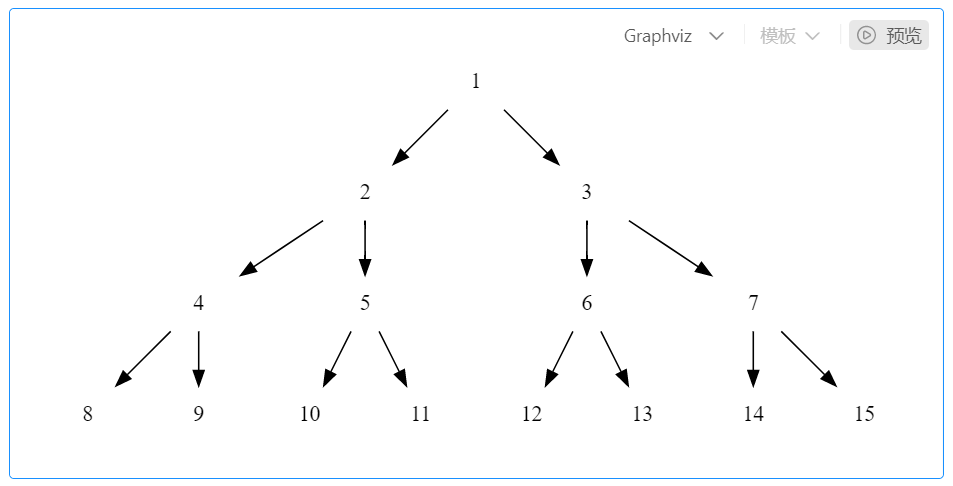#### 3.2.9 Inheritance

``````digraph UML {

node[fontname = "Courier New", fontsize = 10, shape = record];
edge[fontname = "Courier New", fontsize = 10, arrowhead = "empty"];

Car[label = "{Car | v : float\nt : float | run() : float}"]

subgraph clusterSome{
bgcolor = "yellow";
Bus[label = "{Bus | | carryPeople() : void}"];
Bike[label = "{bike | | ride() : void}"];
}
Bus -> Car
Bike -> Car
}``````

Effect picture: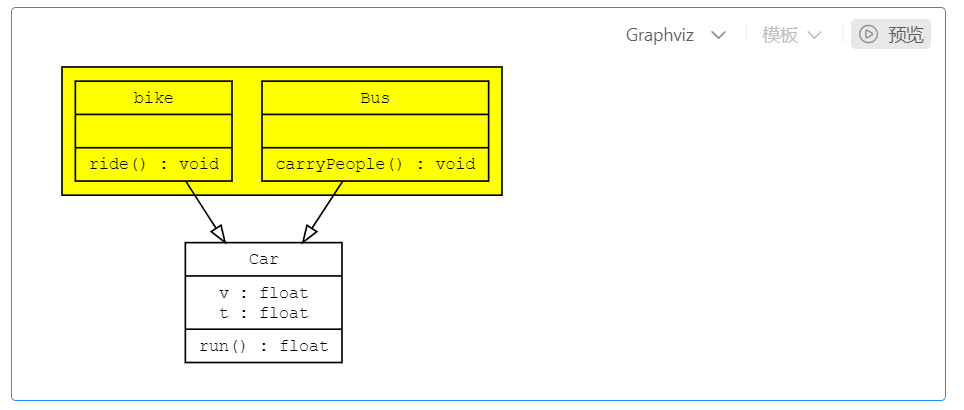#### 3.2.10 Timing Diagram

``````digraph time {

rankdir = "LR";
node[shape = "point", width = 0, height = 0];
edge[arrowhead = "none", style = "dashed"];

{
rank = "same"
edge[style = "solided"];
APP[shape = "plaintext"];
APP -> step00 -> step01 -> step02 -> step03 -> step04 -> step05;
}

{
rank="same";
edge[style="solided"];
SDK[shape="plaintext"];
SDK -> step10 -> step11 -> step12 -> step13 -> step14 -> step15;
}
{
rank="same";
edge[style="solided"];
AliPay[shape="plaintext"];
AliPay -> step20 -> step21 -> step22 -> step23 -> step24 -> step25;
}
{
rank="same";
edge[style="solided"];
Server[shape="plaintext"];
Server -> step30 -> step31 -> step32 -> step33 -> step34 -> step35;
}

step00 -> step10 [label="sends order info", arrowhead="normal"];
step11 -> step21 [label="open AliPay", arrowhead="normal"];
step22 -> step12 [label="pay success", arrowhead="normal"];
step13 -> step03 [label="pay success", arrowhead="normal"];
step24 -> step34 [label="pay success", arrowhead="normal"];
}``````

Effect picture: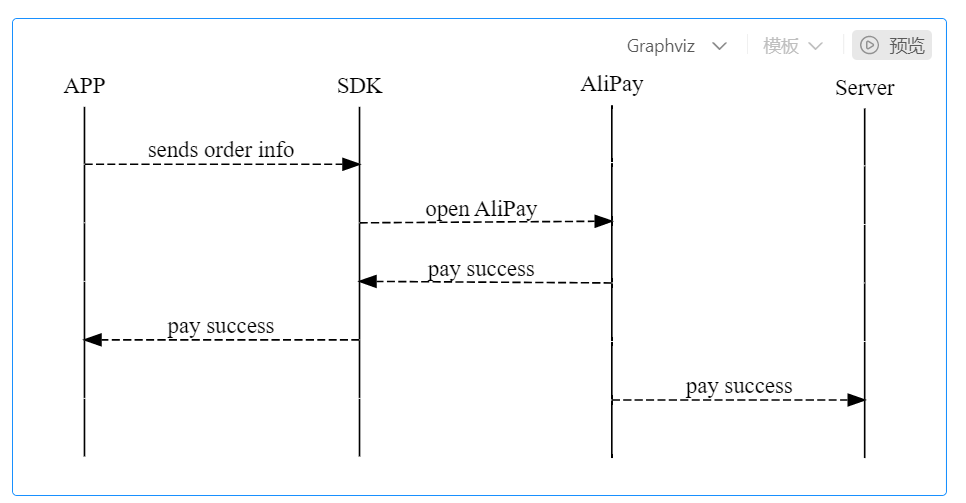## 4. Reference

Attachment: If there are any deficiencies or omissions, please point out. I can learn from them and supplement them.